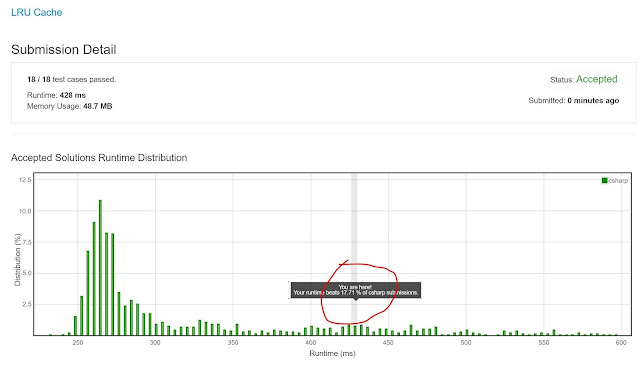## Posts

Showing posts from August, 2019

### Insert Delete GetRandom O(1) - Duplicates allowed (Hard)Problem is here (#424 solved in my list):  https://leetcode.com/problems/insert-delete-getrandom-o1-duplicates-allowed/ Design a data structure that supports all following operations in  average   O(1)  time. Note: Duplicate elements are allowed. insert(val) : Inserts an item val to the collection. remove(val) : Removes an item val from the collection if present. getRandom : Returns a random element from current collection of elements. The probability of each element being returned is  linearly related  to the number of same value the collection contains. Example: // Init an empty collection. RandomizedCollection collection = new RandomizedCollection(); // Inserts 1 to the collection. Returns true as the collection did not contain 1. collection.insert(1); // Inserts another 1 to the collection. Returns false as the collection contained 1. Collection now contains [1,1]. collection.insert(1); // Inserts 2 to the collection, returns true. Collection now contains [1,1,2]

### Find Dupes: An O(NLogN) SolutionHere is the problem (#423 in my solved list):  https://leetcode.com/problems/find-the-duplicate-number/ Given an array  nums  containing  n  + 1 integers where each integer is between 1 and  n  (inclusive), prove that at least one duplicate number must exist. Assume that there is only one duplicate number, find the duplicate one. Example 1: Input: [1,3,4,2,2] Output: 2 Example 2: Input: [3,1,3,4,2] Output: 3 Note: You  must not  modify the array (assume the array is read only). You must use only constant,  O (1) extra space. Your runtime complexity should be less than  O ( n 2 ). There is only one duplicate number in the array, but it could be repeated more than once. The key here are the two statements (conditions) in yellow above: no extra space, and some time complexity better than O(N^2). The first option that came to my mind then would be an O(NLogN): sort the array, and do a linear scan checking which two adjacent elements are the same, and when you find i

### Baseball Game: A Stack SolutionYou can find the problem here (#422 in my solved list): https://leetcode.com/problems/baseball-game/ You're now a baseball game point recorder. Given a list of strings, each string can be one of the 4 following types: Integer  (one round's score): Directly represents the number of points you get in this round. "+"  (one round's score): Represents that the points you get in this round are the sum of the last two  valid  round's points. "D"  (one round's score): Represents that the points you get in this round are the doubled data of the last  valid  round's points. "C"  (an operation, which isn't a round's score): Represents the last  valid  round's points you get were invalid and should be removed. Each round's operation is permanent and could have an impact on the round before and the round after. You need to return the sum of the points you could get in all the rounds. Example 1: Input: ["

### Compare Strings by Frequency of the Smallest CharacterKind of a weird problem, but anywho, here it is:  https://leetcode.com/problems/compare-strings-by-frequency-of-the-smallest-character/ Approach is simple: Build a frequency function (linear in the length of the input string) Build the frequency arrays for both queries and words Sort frequency words At this point you could do a Binary Search (ideal) or use a search for the first instance where the frequency query is larger than the frequency word, and halt (that's what I did) Worked reasonably well. Code is below, cheers, ACC. public class Solution { public int[] NumSmallerByFrequency(string[] queries, string[] words) { int[] queriesNumbers = new int[queries.Length]; int[] wordsNumbers = new int[words.Length]; for (int i = 0; i < queries.Length; i++) { queriesNumbers[i] = Frequency(queries[i]); } for (int i = 0; i < words.Length; i++) { wordsNumbers[i] = Frequency(words[i

### Least Recently Used (LRU) Cache, by LeetCodeI've solved an LRU from DailyCodingProblem before, but finally stumbled on one by LeetCode (#418 in my solved list), here it is:  https://leetcode.com/problems/lru-cache/ Design and implement a data structure for  Least Recently Used (LRU) cache . It should support the following operations:  get  and  put . get(key)  - Get the value (will always be positive) of the key if the key exists in the cache, otherwise return -1. put(key, value)  - Set or insert the value if the key is not already present. When the cache reached its capacity, it should invalidate the least recently used item before inserting a new item. The cache is initialized with a  positive  capacity. Follow up: Could you do both operations in  O(1)  time complexity? Example: LRUCache cache = new LRUCache( 2 /* capacity */ ); cache.put(1, 1); cache.put(2, 2); cache.get(1); // returns 1 cache.put(3, 3); // evicts key 2 cache.get(2); // returns -1 (not found) cache.put(4, 4); // evicts key

### Custom Sort String: Modified BubbleSortProblem is here (#416th in my solved list):  https://leetcode.com/problems/custom-sort-string/ S  and  T  are strings composed of lowercase letters. In  S , no letter occurs more than once. S  was sorted in some custom order previously. We want to permute the characters of  T  so that they match the order that  S was sorted. More specifically, if  x  occurs before  y  in  S , then  x  should occur before  y  in the returned string. Return any permutation of  T  (as a string) that satisfies this property. Example : Input: S = "cba" T = "abcd" Output: "cbad" Explanation: "a", "b", "c" appear in S, so the order of "a", "b", "c" should be "c", "b", and "a". Since "d" does not appear in S, it can be at any position in T. "dcba", "cdba", "cbda" are also valid outputs. Note: S  has length at most  26 , and no chara